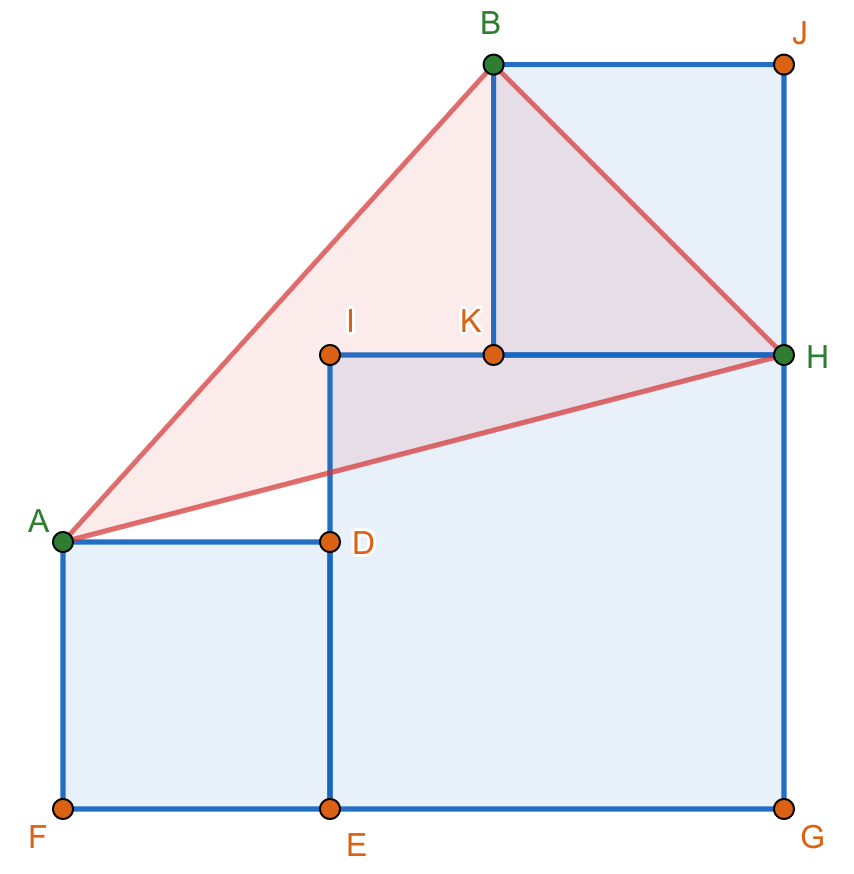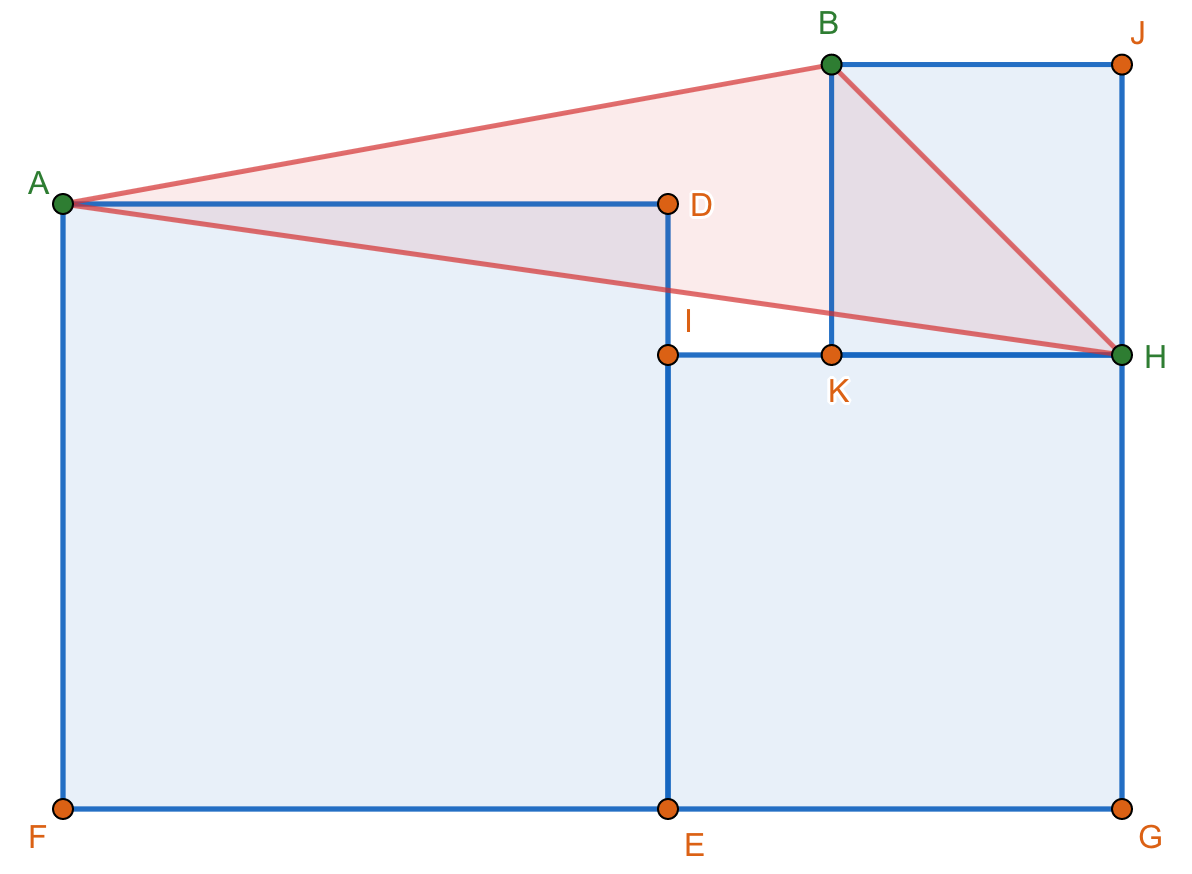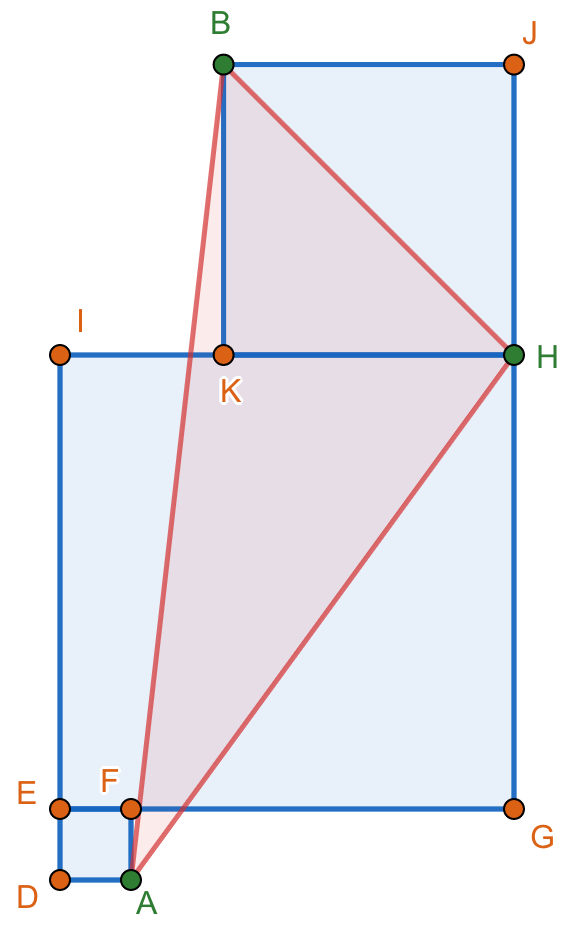# Constant Area of Triangle ABCFrom the figures shown below. Squares EGHI and KHJB are fixed. Show that the area of triangle ABH is constant regardless of the dimensions of square ADEF.

Figure 1Figure 2Figure 3Figure 4### I don't really know how to

I don't really know how to prove this one sir. I just noticed when i substituted values, by taking line BH as the base, we will be having the same height in all triangles which we all know that if two triangles have the same base and height, their areas will be the same if i will not be mistaken.### Yes you are right. What you

Yes you are right. What you have in mind is the correct proof. All you need to do is to express it, the best is if you can articulate it through words and equations.

### 1. If we draw a line that

1. The diagonals of a square are perpendicular to each other.

2. If we draw a line extending diagonal AE of square AFED, we can see that vertex A is still going to land on this line no matter what the size of square AFED.

3. We can also see that diagonal AE is perpendicular to diagonal BH since their bases are parallel on the horizon lets say x.

Having these in mind and setting BH as the base of the triangle, and since vertex A can also land on vertex E, we can say that Area ABH=1/2 (BH)(EG2+GH2)1/2

• Mathematics inside the configured delimiters is rendered by MathJax. The default math delimiters are $$...$$ and $...$ for displayed mathematics, and $...$ and $...$ for in-line mathematics.